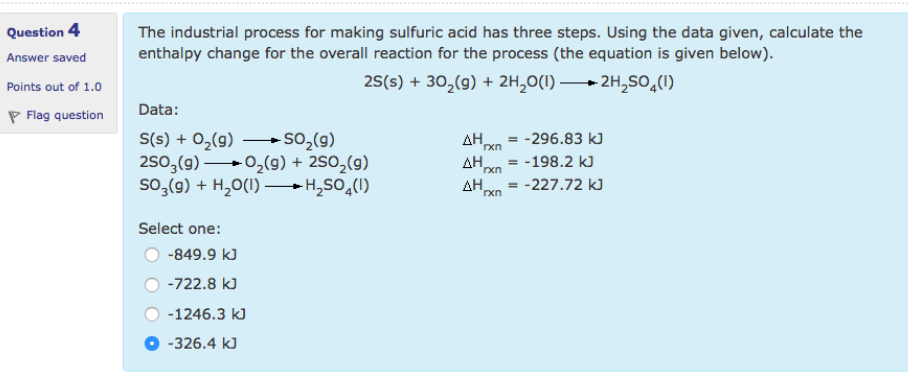Chemistry Practice Problems Hess's Law Practice Problems Solution: The industrial process for making sulfuric acid ha...

# Solution: The industrial process for making sulfuric acid has three steps. Using the data given, calculate the enthalpy change for the overall reaction for the process (the equation is given below).2S(s) + 3O2(g) rightarrow 2H2SO4(l) Data:S(s) + O2(g) → SO(g) Δ Hrxn = -296.83 kJ2SO3(g) → O2(g) + 2SO2(g) ΔHrxn = - 198.2 kJSO3(g) + H2O(l) → H2SO4(l) ΔHrxn = - 227.72 kJSelect one:•-849.9 kJ•-722.8 kJ•-1246.3 kJ•-326.4 kJ

###### Problem

The industrial process for making sulfuric acid has three steps. Using the data given, calculate the enthalpy change for the overall reaction for the process (the equation is given below).

2S(s) + 3O2(g) rightarrow 2H2SO4(l)

Data:
S(s) + O2(g) → SO(g) Δ Hrxn = -296.83 kJ
2SO3(g) → O2(g) + 2SO2(g) ΔHrxn = - 198.2 kJ
SO3(g) + H2O(l) → H2SO4(l) ΔHrxn = - 227.72 kJ

Select one:

•-849.9 kJ
•-722.8 kJ
•-1246.3 kJ
•-326.4 kJView Complete Written Solution

Hess's Law

Hess's Law

#### Q. Consider the following hypothetical reactions:A → B          ΔH = +20kJ B → C         ΔH = +66kJ Use Hess's law to calculate the enthalpy change for t...

Solved • Tue May 29 2018 17:16:20 GMT-0400 (EDT)

Hess's Law

#### Q. Given the following data: N2(g) + O2(g) → 2NO(g),          ΔH = +180.7 kJ 2NO(g) + O2(g) → 2NO2(g),     ΔH = -113.1 kJ 2N2O(g) → 2N2(g) + O2(g),      ...

Solved • Tue May 29 2018 17:08:19 GMT-0400 (EDT)

Hess's Law

#### Q. From the enthalpies of reaction 2C(s) + O2(g) → 2CO(g)                             ΔH = -221.0 kJ 2C(s) + O2(g) + 4H2(g) → 2CH3OH(g)       ΔH = -402.4...

Solved • Tue May 29 2018 16:31:52 GMT-0400 (EDT)

Hess's Law

#### Q. Nitroglycerine is a powerful explosive that forms four different gases when detonated:2 C3H5(NO3)3(l) → 3 N2(g) + 1/2 O2(g) + 6 CO2(g) + 5 H2O(g)Calcu...

Solved • Mon May 21 2018 08:22:48 GMT-0400 (EDT)